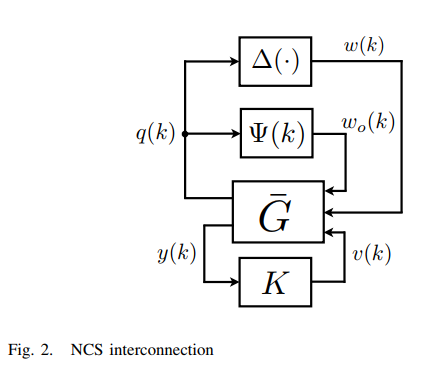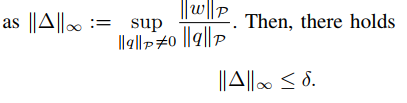# General Question

Hi, I have a problem which i don’t know how can i model the network control system with matlab as m.file. in this problem all of system are in discrete time domain: A1 = [1.1 1;0 1.2] ; B1 = [0 ;1] ; C1 = [1 0] G¯ :x(k + 1) = Ax(k) + Bµv(k) + Bµw(k)+Bµwo(k); y(k) = Cx(k); and controller achieved from an LMI condition and is : K : xk(k + 1) =Ak xk(k) +Bk y(K) v(K) = Ck xk(K) + Dk y(k); K1 = −1.2458(z − 1:012)(z − 0:5753)/(z − 0:5478)(z + 0:997)

uncertainty block is : ∆(·) is a nonlinear operator. We assume that ∆(0) = 0 is the unique equilibrium point and define the infinity norm of ∆(·) as : And sai(k) is Ψ(k) = (Φ(k) − µ)/µ which E{Φ(k)} = µ and µ (0; 1] and Φ(k) is where Φ(k) is an i.i.d.(independent and identically distributed) Bernoulli process.